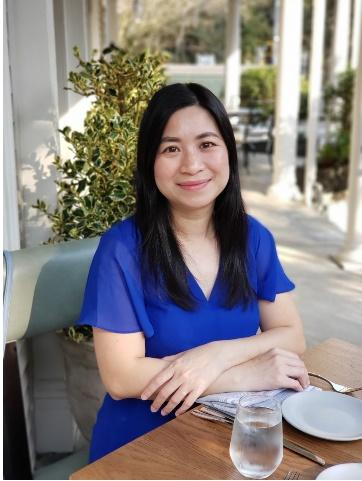Dr. Ha Nguyen is an Assistant Professor of Mathematics Education at Georgia Southern University. She is interested in exploring (1) the teaching and learning of mathematics for preservice and inservice teachers and undergraduate students, (2) teaching mathematics for social justice, and (3) STEM teaching and learning. She earned her Ph.D. in mathematics from Emory University.
 Using Virtual Tools to Explore Volume Formulas for Prisms, Cylinders, Pyramids, and Cones I teach mathematics content courses for K-8 preservice teachers. Like many educators, when COVID-19 hit and classes went online or hybrid, I had to find new ways to engage students in learning. In this paper, I will share how I adapted my lesson on exploring volume formulas of prisms, cylinders, pyramids, and cones to support learning in various classroom settings such as in-person with social distancing and in Zoom. Even though my students are college students, the content and activities can also be used in K-8 classes. Context During a normal semester, my classes tend to be fairly active. Students are put into groups of four or five to discuss tasks, and then their answers and strategies are purposefully selected to share with the whole class. During the pandemic, the learning modalities include in-person groups of students in the classroom with social distancing and students Zooming in from outside of class. To accommodate this change in learning modes, I used interactive tools and Desmos.             Desmos is a free activity builder platform. Each Desmos lesson is designed with a series of screens that walk each student through interactive activities, where students can participate with their phones or laptops. As students move through the inquiry-based activities on Desmos, I can observe their progress and understanding on the dashboard, which displays student responses with their names being anonymized by mathematician names. If I notice a misconception performed by students, I pause the activity and discuss it with the whole class. Volumes To investigate volume formulas for pyramids, prisms, cones, and cylinders, students were set to explore the following in order: 1) Meaning of volume, 2) Volume formula for prisms and cylinders, and 3) Volume formula for pyramids and cones. Meaning of Volume Before exploring volume formulas of three-dimensional objects, students must understand what volume means first. To do this, we used the interactive Cubes tool (Illuminations, n.d.) (see Figure 1), which shows a rectangular prism of 4 unit-by-5-unit-by-3-unit, and had a class discussion. (Note that the dimensions of the rectangular prism can be customized using the setting tools on the top right corner.) Figure 1 Cubes Activity in Illuminations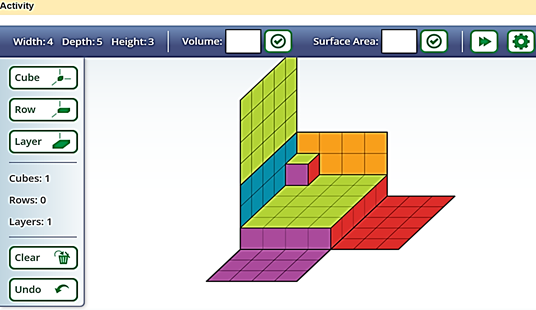The tool allows us to drop a 1-unit-by-1-unit-by-1-unit cube (unit cubes), or a row of the unit cubes, or a layer of the unit cubes into the rectangular box. I clicked on the flattened sides of the box to show students what the box looks like when the sides are folded up. Students were reminded that area means the number of square units it takes to cover a two-dimensional shape without any gaps or overlaps. They were then asked to define what volume is. After seeing unit cubes being dropped into the rectangular box, students often had no difficulty linking area concepts to volume and quickly answer, “Volume is the number of cubic units it takes to fill the solid.” Volume formula for Prisms and Cylinders Once students understood the meaning of volume, they made sense of the volume formula for prisms and cylinders through the following whole-class discourse: Teacher: We like to be efficient when we can. If we don’t want to fill this rectangular prism with one 1-unit-by-1-unit-by-1-unit cube, or unit cubes, at a time and want to speed up the process, what can we do? Yooni: We can use a row instead. Teacher: Great! Thanks, Youni. For this particular rectangular prism (of dimensions 4-unit-by-5-unit-by-3-unit), how many unit cubes fill one row? Alex: 4 cubes. Teacher: That’s correct! Thanks, Alex. Instead of filling the box by rows, what else can we do? Huang: We can fill the whole bottom. Teacher: That’s a great idea, Huang! So how many unit cubes we need to fill the bottom layer and why? Justin: There are 4 unit cubes in 1 row, and there are 5 rows. So total 20 unit cubes. Teacher: Excellent, Justin! So how many layers will we need to fill the entire box? Sophie: I think 3 layers because I see the blue vertical side goes up to 3, and that’s where you close the box. Teacher: That was great, Sophie! Now, 3 is the value of one of the dimensions of this rectangular prism. What is the name of that dimension? Lily: Is that the height of the prism? Teacher: That’s correct, Lily! To summarize, we can either fill the entire rectangular box with one unit cube at the time, or we fill the box with one layer at the time, and the number of the layers depends on the height of the box. After the whole class discussion, students came to realize, as seen in Beckmann (2017), that  Volume of a prism = (Number of unit cubes in 1 layer) * (Number of layers) Equivalently, Volume of a prism = (Area of the base) * (Height) From there, students related prims to cylinders and saw that they can follow a similar process to fill a cylinder; that is, to fill the cylinder with one layer first, and then stack up the layers to reach the other base of the cylinder. Thus, the volume formula for prisms also works for cylinders. For a circular cylinder, the base is a circle. Therefore, the area of the base, in this case, is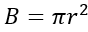where B is the area of the base, and r is the radius of the base. Volume formula for pyramids and cones I adopted Torres’s (n.d.) Desmos task on Volume formula for pyramids and cones, which was based on the work and videos of Pearce (n.d.). In particular, students were given three sets of three-act mathematics tasks (Meyer, 2011; Fletcher, 2016) including comparisons between 1) a rectangular prism and pyramid, 2) a triangular prism and pyramid, and 3) a right circular cylinder and cone. A three-act mathematics task was developed by Meyer for secondary school students, which Fletcher and other educators modified for elementary school students. A three-act task consists of three distinct parts: Act 1, Act 2, and Act 3. In Act 1, students view a photograph or video and discuss what they notice and wonder. Then in Act 2, students work on finding solutions to the problem. Finally, in Act 3, students discuss their mathematical models to the problems. A description of how Task 1: Rectangular Prism versus Rectangular Pyramid was implemented will be given in the following section. The other two tasks (comparing a triangular prism and pyramid, and comparing a right circular cylinder and cone) will be similar. Task 1: Rectangular Prism versus Rectangular Pyramid Act 1: What do you notice? What do you wonder? In this Act 1, students watched a 14-second video showing a rectangular prism and a rectangular pyramid. Then they were asked to type in Desmos what they noticed and wondered. In this one group of students, some noticed that both solids had the same height and base. Some wondered if these solids have the same volume. One wondered the difference in area between the two “shapes.” Another one declared that the pyramid had one-third of the volume of a prism, “regardless of the polygon and height.” Figure 2 displays this group of student responses in Desmos. Notice that their names are being anonymized by mathematician names. Figure 2 Students Noticed and Wondered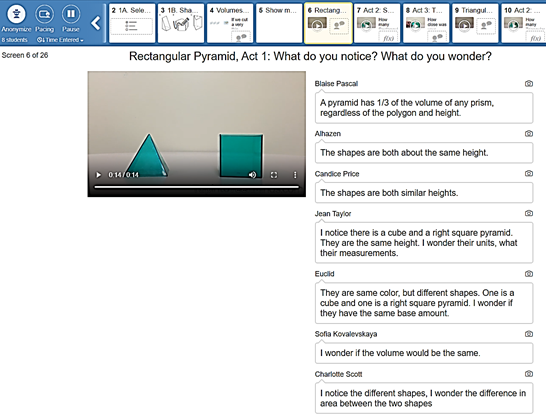Act 2: Some More Information In this Act 2, students watched a 21-second video showing the bases of the rectangular prism and rectangular pyramid side by side. Then water from the fully filled the rectangular pyramid was being poured into the rectangular prism. Students were asked to guess how many rectangular pyramids it would take to fill the rectangular prism. In this group of students, most answered “2” or “3,” while one student said “1.” Figure 3 displays a group of student guesses in Desmos. Figure 3 Student Guesses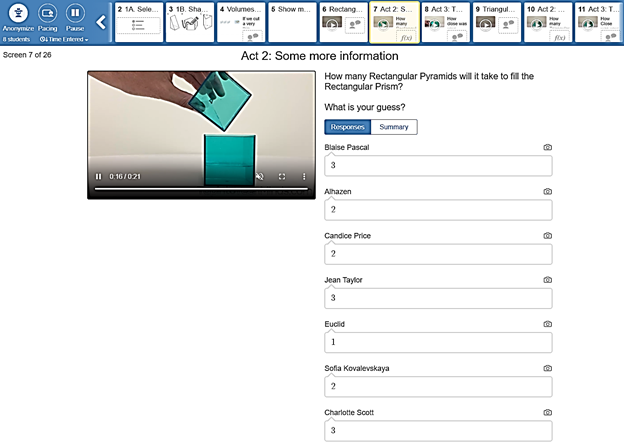Act 3: The Answer In this Act 3, students watched a 40-second video, showing that it took three rectangular pyramids to fill one rectangular prism of the same height and base. We then discussed student guesses in Act 2 and pointed out that one rectangular pyramid did not fill the rectangular prism fully, so the number of rectangular pyramids to fill the rectangular prism should not have been “1.” This helped strengthen students' ability to judge their guesses and realize whether their guesses would make sense in a context. Figure 4 displays a group of student answers on how close their estimates were. Figure 4 How Close Students’ Estimates Were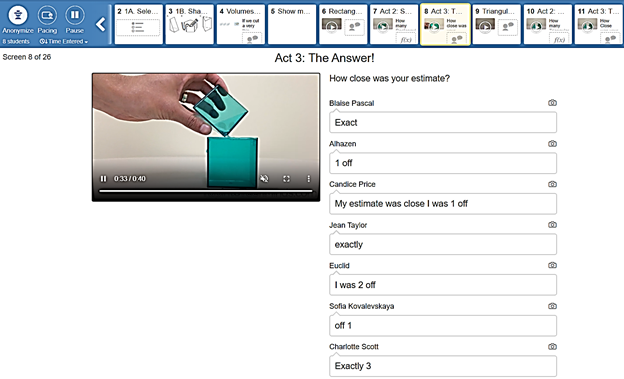Following this task, when comparing a triangular pyramid versus a triangular prism and a circular cone versus a circular cylinder next, students focused more on the volume, height, and bases of each pair of the solids in their notice and wonder, and their guesses became more reasonable. Most immediately wondered if the volume of a triangular prism / circular cylinder is three times the volume of the triangular pyramid / circular cone of the same size base and equal height. All but one student guessed that it took “3” triangular pyramids to fill a triangular prism as well as “3” circular cones to fill a circular cylinder of the same size base and equal heights. They moved on to form the volume formula for pyramids, stating that since the volume of a prism is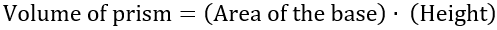and three pyramids of the same base and height fill the prism, the volume of a pyramid is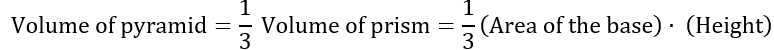. Students came to the same conclusion for the volume formula of circular cones, where the area of the base in this case is the area of a circle. Closing Thoughts During the pandemic when students cannot work in small groups in person and with physical manipulatives in front of them in the classroom, virtual tools such as Illumination Cubes (n.d.) and Desmos tasks can be nice substitutes. Such tools promote active learning and can be used in various classroom settings: in-person with social distancing, in Zoom, as well as in-person during a typical academic year. Figure 5 reveals reflections from a group of students at the end of the volume lesson. They claimed that they understood the concepts, and the activities helped them learn the content, even when geometry confused them before. Others informally shared that it was fun to learn mathematics when there was no pressure to get the “right” answers. By letting students explore concepts on their own in Desmos with their names being anonymized, they feel more comfortable sharing their answers on the screen, learn as a community, and come to discoveries on their own, thereby taking ownership over concepts, which helps them gain deeper understanding. Figure 5 Student Reflections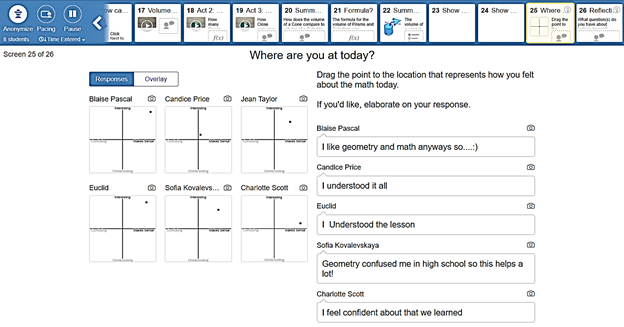References: Beckmann, S. (2017). Mathematics for Elementary Teachers with Activities. 5th ed. New York: Pearson. Fletcher, G. (July 29, 2016). Modeling with Mathematics through Three-Act Tasks. Math Tasks to Talk About. http://www.nctm.org/Publications/TeachingChildren-Mathematics/Blog/Modeling-with-Mathematics-through-Three-Act-Tasks/ Meyer, D. (May 11, 2011). The Three Acts of a Mathematical Story. Dy/Dan (blog). http://blog.mrmeyer.com/2011/the-three-acts-of-a-mathematical-story/ National Council of Teachers of Mathematics. (n.d.). Illuminations. http://illuminations.nctm.org/Activity.aspx?id=4095 Pearce, K. (n.d). Tap Into Teen Minds.  https://tapintoteenminds.com/3act-math/prisms-pyramids/ Torres, M. (n.d.). Volume comparisons with prisms and pyramids, cones and cylinders. https://teacher.desmos.com/activitybuilder/custom/5adce81eed2ada6785169a39 Find a printable PDF version of this article here.